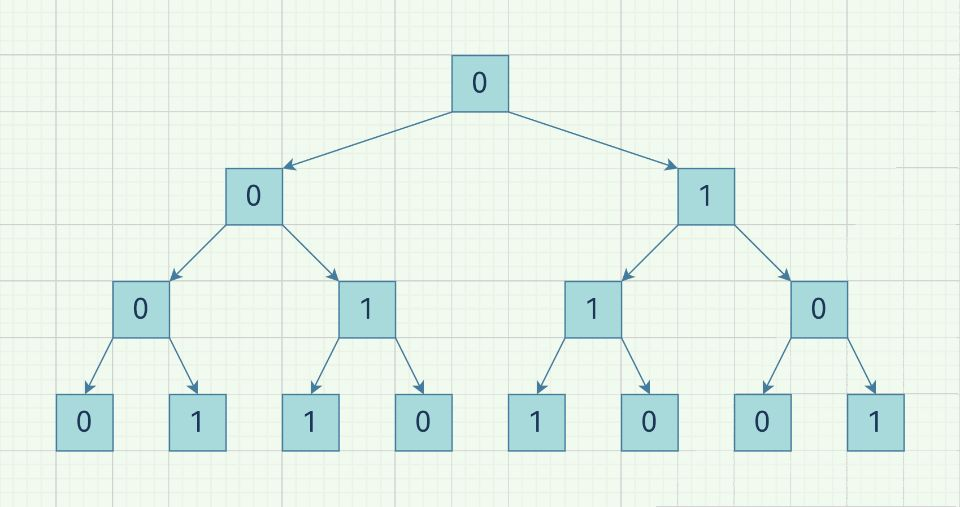## 题目描述

leetcode 779. 第K个语法符号

• 例如，对于 `n = 3` ，第 `1` 行是 `0` ，第 `2` 行是 `01` ，第3行是 `0110`

```输入: n = 1, k = 1

```

```输入: n = 2, k = 1

```

```输入: n = 2, k = 2

```

• `1 <= n <= 30`
• `1 <= k <= 2n - 1`

## 题解——脑筋急转弯：作图理解，c++一行

### 思路